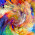### Force - Basic Definition

Force may be defined as an agent which produces or tends to produce, destroy or tends to destroy the motion of a body. A force while acting on a body may

(a) change the motion of a body,
(b) retard the motion of a body,
(c) balance the forces already acting on a body, and
(d) give rise to the internal stresses in a body.

In order to determine the effects of a force acting on a body, we must know the following characteristics of a force:

(i) The magnitude of the force,
(ii) The line of action of the force,
(iii) The nature of the force, i.e. push or pull, and
(iv) The point at which the force is acting.

In M. K. S. system of units, the magnitude of the force is expressed in kilogram-force (briefly written as kgf) and in S.I. system of units, the force is expressed in newtons (briefly written as N). It may be noted that

1 kgf = 9.81 N

1.can we have all the materials in pdf format

2.Nice explanation

3.you are doing great job...a true mechanical engineer..keep it up